# Search Results (44)

View
Selected filters:
• Place Value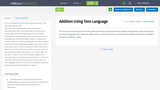Unrestricted Use
CC BY
Rating

This is a modification of the Illustrative Math lesson, "Ford and Logan ...

This is a modification of the Illustrative Math lesson, "Ford and Logan add 45 + 36," https://www.illustrativemathematics.org/content-standards/tasks/2068. This lesson was used with a tier 3 fourth grade intervention math group to help students develop their understanding of place value and use of tens language when adding two digit numbers. I modified it to meet the students' needs by adding ways two other students (Sarah and Tammy) solved the problem: Sarah said, "I know 4 tens plus 3 tens is 7 tens; 5 ones plus 6 ones is eleven ones which is 1 ten and 1 one so 4 tens plus 3 tens plus 1 tens is 8 tens and the one left so the answer is 81." Tammy said, " 45 plus 30 is 75, plus 6 more is 81." Students analyzed and discussed all four methods and compared them to their own methods. I then had students practice using the tens and ones language (like Sarah) for some more addition problems without using place value blocks or models.

Subject:
Mathematics
Material Type:
Author:
MEGAN HINDE
08/02/2019Restricted Use
Rating

In this online game, students will be building numbers using Base 10 ...

In this online game, students will be building numbers using Base 10 blocks.  Students decide if they want to select ones, tens, or hundreds.  Students also get to choose if they want to read the number, listen to the number, or if they want to count the blocks given and deterine the number being represented.

Subject:
Mathematics
Material Type:
Game
Interactive
Provider:
ABCya.com
12/21/2016Conditional Remix & Share Permitted
CC BY-NC-SA
Rating

This instructional task requires students to figure out word problems that require ...

This instructional task requires students to figure out word problems that require thinking in base 10.

Subject:
Mathematics
Numbers and Operations
Material Type:
Activity/Lab
Provider:
Illustrative Mathematics
Provider Set:
Illustrative Mathematics
Author:
Illustrative Mathematics
05/01/2012Conditional Remix & Share Permitted
CC BY-NC-SA
Rating

In this task students determine the number of hundreds, tens and ones ...

In this task students determine the number of hundreds, tens and ones that are necessary to write equations when some digits are provided. Students must, in some cases, decompose hundreds to tens and tens to ones.

Subject:
Mathematics
Numbers and Operations
Material Type:
Activity/Lab
Provider:
Illustrative Mathematics
Provider Set:
Illustrative Mathematics
Author:
Illustrative Mathematics
05/01/2012Educational Use
Rating

This activity provides many ideas for celebrating the 100th Day of school.

Subject:
Fine Arts
Numbers and Operations
Material Type:
Lesson Plan
Provider:
Utah Education Network
11/01/2017Rating

In this Flash game, students may use a variety of activities to ...

In this Flash game, students may use a variety of activities to practice counting, collecting, exchanging, and making change for coins. Coin representations may show their amounts or not; coins may also be represented by coin tiles on a 10x10 grid, to help visualize their values. The five activities are: count, in which students determine the value of a given set of coins; collect, in which students choose coins to represent a given value; exchange, in which students use the smallest number of coins to represent a given value; change from coins, in which students are given a set of coins and asked how much change would be for a dollar (essentially counting up); and change from value, in which students are given a price owed (from a dollar) and asked to make change. There is a "bank" at the lower left side of the applet in which coins may be exchanged for other coins of the same value.

Subject:
Mathematics
Material Type:
Interactive
Lesson Plan
Provider:
National Council of Teachers of Mathematics
Provider Set:
Illuminations
11/05/2011Conditional Remix & Share Permitted
CC BY-NC-SA
Rating

In this task students are required to compare numbers that are identified ...

In this task students are required to compare numbers that are identified by word names and not just digits. The order of the numbers described in words are intentionally placed in a different order than their base-ten counterparts so that students need to think carefully about the value of the numbers.

Subject:
Mathematics
Numbers and Operations
Material Type:
Activity/Lab
Provider:
Illustrative Mathematics
Provider Set:
Illustrative Mathematics
Author:
Illustrative Mathematics
05/01/2012Conditional Remix & Share Permitted
CC BY-NC-SA
Rating

In this task students are required to compare numbers that are identified ...

In this task students are required to compare numbers that are identified by word names and not just digits. The order of the numbers described in words are intentionally placed in a different order than their base-ten counterparts so that students need to think carefully about the value of the numbers.

Subject:
Mathematics
Numbers and Operations
Material Type:
Activity/Lab
Provider:
Illustrative Mathematics
Provider Set:
Illustrative Mathematics
Author:
Illustrative Mathematics
05/01/2012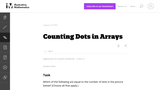Conditional Remix & Share Permitted
CC BY-NC-SA
Rating

Students who work on this task will benefit in seeing that given ...

Students who work on this task will benefit in seeing that given a quantity, there is often more than one way to represent it, which is a precursor to understanding the concept of equivalent expressions.

Subject:
Mathematics
Numbers and Operations
Material Type:
Activity/Lab
Provider:
Illustrative Mathematics
Provider Set:
Illustrative Mathematics
Author:
Illustrative Mathematics
05/01/2012Conditional Remix & Share Permitted
CC BY-NC-SA
Rating

This is an instructional task related to deepening place-value concepts. The important ...

This is an instructional task related to deepening place-value concepts. The important piece of knowledge upon which students need to draw is that 10 tens is 1 hundred.

Subject:
Mathematics
Numbers and Operations
Material Type:
Activity/Lab
Provider:
Illustrative Mathematics
Provider Set:
Illustrative Mathematics
Author:
Illustrative Mathematics
05/01/2012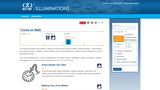Rating

This unit contains two lessons which help students develop number sense through ...

This unit contains two lessons which help students develop number sense through activities involving collection, representation, and analysis of data. Students also practice reading and writing large numbers and develop estimation skills. In Lesson 1, Every Breath You Take, students estimate the number of breaths taken during a specified time, experiment, and display real-life data. In Lesson 2, Making Your First Millions, students develop the concept of a million by working with smaller numerical units, such as blocks of 10 or 100, and then expanding the idea by multiplication or repeated addition. They analyze situations and identify patterns that will enable them to develop the concept of large numbers. Each lesson includes student activity sheets, an instructional plan, and extensions.

Subject:
Mathematics
Material Type:
Interactive
Lesson Plan
Provider:
National Council of Teachers of Mathematics
Provider Set:
Illuminations
Author:
William R. Speer and Daniel J. Brahier
11/05/2000Rating

This place value and problem solving lesson focuses on forming 3-digit address ...

This place value and problem solving lesson focuses on forming 3-digit address numbers to meet specific requirement. The lesson provides an opportunity for learners to use the problem-solving strategies of looking for patterns and establishing an organized list. Students also learn that careful reading of information and understanding of mathematical language are important to finding appropriate solutions.

Subject:
Mathematics
Material Type:
Interactive
Lesson Plan
Provider:
National Council of Teachers of Mathematics
Provider Set:
Illuminations
11/05/2014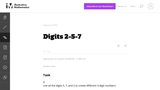Conditional Remix & Share Permitted
CC BY-NC-SA
Rating

This task helps students understand the relationship between digit placement and the ...

This task helps students understand the relationship between digit placement and the value of a number.

Subject:
Mathematics
Numbers and Operations
Material Type:
Activity/Lab
Provider:
Illustrative Mathematics
Provider Set:
Illustrative Mathematics
Author:
Illustrative Mathematics
05/01/2012Rating

In this task, a group of children sit around a table. Without ...

In this task, a group of children sit around a table. Without peeking, they figure out how many feet are under the table. They can use mathematical tools, such as cubes or drawings, that will help them.

I extended off of this to add it to our development of place value, groups of ten, and extra ones. I added an additional material of a tens frame to help students see the grouping of ten and extra ones. After we worked through all the steps (1-6) I added an additional step of scenarios where additional students were added to continue working with larger numbers with groupings of tens and extra ones. As well, students who needed to work with counting on from larger two digit numbers, this activity also helped to increase that knowledge.

Subject:
Mathematics
Material Type:
Author:
C. Confer
You Cubed
04/27/2018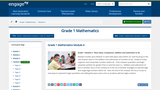Conditional Remix & Share Permitted
CC BY-NC-SA
Rating

Module 4 builds upon Module 2s work with place value within 20, ...

Module 4 builds upon Module 2s work with place value within 20, now focusing on the role of place value in the addition and subtraction of numbers to 40.  Students study, organize, and manipulate numbers within 40.  They compare quantities and begin using the symbols for greater than (>) and less than (<).  Addition and subtraction of tens is another focus of this module as is the use of familiar strategies to add two-digit and single-digit numbers within 40.  Near the end of the module, the focus moves to new ways to represent larger quantities and adding like place value units as students add two-digit numbers.

Subject:
Numbers and Operations
Material Type:
Module
Provider:
New York State Education Department
Provider Set:
EngageNY
09/25/2013Conditional Remix & Share Permitted
CC BY-NC-SA
Rating

Module 1 sets the foundation for students to master the sums and ...

Module 1 sets the foundation for students to master the sums and differences to 20 and to  subsequently apply these skills to fluently add one-digit to two-digit numbers at least through 100 using place value understandings, properties of operations and the relationship between addition and subtraction.

Subject:
Numbers and Operations
Material Type:
Module
Provider:
New York State Education Department
Provider Set:
EngageNY
05/11/2013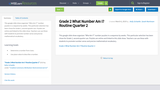Conditional Remix & Share Permitted
CC BY-NC
Rating

This google slide show organizes "Who Am I?" number puzzles in a ...

This google slide show organizes "Who Am I?" number puzzles in a sequence by weeks. This particular selection has been chose for Grade 2, second quarter use. Puzzles are online and linked to this slide show. Teachers can use these with students to promote number sense and precise mathematical vocabulary.

Subject:
Mathematics
Material Type:
Activity/Lab
Author:
Sarah Martinsen
Kelly Schaefer
08/02/2019Conditional Remix & Share Permitted
CC BY-NC
Rating

This google slide show organizes "What Am I?" number puzzles in a ...

This google slide show organizes "What Am I?" number puzzles in a sequence by weeks. This particular selection has been chose for second grade, third quarter use. Teachers can use these with students to promote number sense and precise mathematical vocabulary.

Subject:
Mathematics
Material Type:
Activity/Lab
Author:
Sarah Martinsen
Kelly Schaefer
08/02/2019Conditional Remix & Share Permitted
CC BY-NC
Rating

This google slide show organizes place value exercises in a sequence by ...

This google slide show organizes place value exercises in a sequence by weeks. Teachers use the slide show with students to promote finding structure and adding and subtracting ones and tens.

Subject:
Mathematics
Material Type:
Activity/Lab
Author:
Stacy Ottman
Kelly Schaefer
08/02/2019Conditional Remix & Share Permitted
CC BY-NC-SA
Rating

In this 25-day module of Grade 4, students extend their work with ...

In this 25-day module of Grade 4, students extend their work with whole numbers.  They begin with large numbers using familiar units (hundreds and thousands) and develop their understanding of millions by building knowledge of the pattern of times ten in the base ten system on the place value chart (4.NBT.1).  They recognize that each sequence of three digits is read as hundreds, tens, and ones followed by the naming of the corresponding base thousand unit (thousand, million, billion).

Subject:
Numbers and Operations
Material Type:
Module
Provider:
New York State Education Department
Provider Set:
EngageNY
05/11/2013Conditional Remix & Share Permitted
CC BY-NC-SA
Rating

In Module 2 students apply patterns of the base ten system to ...

In Module 2 students apply patterns of the base ten system  to mental strategies and a sequential study of multiplication via area diagrams and the distributive property leading to fluency with the standard algorithm.  Students move from whole numbers to multiplication with decimals, again using place value as a guide to reason and make estimations about products. Multiplication is explored as a method for expressing equivalent measures in both whole number and decimal forms.  A similar sequence for division begins concretely with number disks as an introduction to division with multi-digit divisors and leads student to divide multi-digit whole number and decimal dividends by two-digit divisors using a vertical written method.  In addition, students evaluate and write expressions, recording their calculations using the associative property and parentheses.  Students apply the work of the module to solve multi-step word problems using multi-digit multiplication and division with unknowns representing either the group size or number of groups.  An emphasis on the reasonableness of both products and quotients, interpretation of remainders and reasoning about the placement of decimals draws on skills learned throughout the module, including refining knowledge of place value, rounding, and estimation.

Subject:
Numbers and Operations
Material Type:
Module
Provider:
New York State Education Department
Provider Set:
EngageNY
07/05/2013Rating

This intereactive Flash applet helps children learn grouping, tally marks, place value, ...

This intereactive Flash applet helps children learn grouping, tally marks, place value, addition, and subtraction. Students help the alien spaceship move cows into corrals by counting by 5s and 10s. They also can apply those grouping skills to practice adding and subtracting two-digit numbers with regrouping. Audio cues and prompts reinforce the user's actions and facilitate counting and the development of math language.

Subject:
Mathematics
Material Type:
Interactive
Lesson Plan
Provider:
National Council of Teachers of Mathematics
Provider Set:
Illuminations
11/05/2000Conditional Remix & Share Permitted
CC BY-NC-SA
Rating

This task gives children an opportunity to subtract a three-digit number including ...

This task gives children an opportunity to subtract a three-digit number including a zero that requires regrouping. The solutions show how students can solve this problem before they have learned the traditional algorithm.

Subject:
Mathematics
Numbers and Operations
Material Type:
Activity/Lab
Provider:
Illustrative Mathematics
Provider Set:
Illustrative Mathematics
Author:
Illustrative Mathematics
10/12/2012Conditional Remix & Share Permitted
CC BY-NC
Rating

This google slide show organizes "What Am I?" number puzzles in a ...

This google slide show organizes "What Am I?" number puzzles in a sequence by weeks. This particular selection has been chose for Kindergarten, third quarter use. Teachers can use these with students to promote number sense and precise mathematical vocabulary.

Subject:
Mathematics
Material Type:
Activity/Lab
Author:
Katie Campbell
Kelly Schaefer
08/02/2019Conditional Remix & Share Permitted
CC BY-NC-SA
Rating

This word problems helps students learn to build the biggest three-digit number ...

This word problems helps students learn to build the biggest three-digit number given any three numbers between 0 and 9 to use as digits.

Subject:
Mathematics
Numbers and Operations
Material Type:
Activity/Lab
Provider:
Illustrative Mathematics
Provider Set:
Illustrative Mathematics
Author:
Illustrative Mathematics
05/01/2012Rating

In this 6-lesson unit students develop concepts of number, relationships between numbers, ...

In this 6-lesson unit students develop concepts of number, relationships between numbers, and equality. They make groups of 10 to 20 objects, connect number names to the groups, compose and decompose numbers, and use numerals to record the size of a group. Each lesson includes visual, auditory, and kinesthetic activities, as well as student activity sheets, questions for teachers and students, ideas for assessment and extensions, and links to electronic applets.

Subject:
Mathematics
Material Type:
Interactive
Lesson Plan
Provider:
National Council of Teachers of Mathematics
Provider Set:
Illuminations
Author:
Grace M. Burton - Towards A Good Beginning: Teaching Early Childhood Mathematics. Menlo Park, CA: Addison-Wesley, 1985
11/05/2000Rating

In this math meets engineering activity, learners make paper airplanes and explore ...

In this math meets engineering activity, learners make paper airplanes and explore attributes related to increasing flight distances. Each learner collects data from three flights of the airplane and finds the median distance. Learners then collect, organize, display, and interpret the median distances for the group in a stem-and-leaf plot. This lesson guide includes questions for learners, assessment options, extensions, and reflection questions.

Subject:
Mathematics
Material Type:
Interactive
Lesson Plan
Provider:
National Council of Teachers of Mathematics
Provider Set:
Illuminations
Author:
National Council of Teachers of Mathematics
NCTM Illuminations
Thinkfinity/Verizon Foundation
11/09/2012Conditional Remix & Share Permitted
CC BY-NC-SA
Rating

This task is for students who know how to use base 10 ...

This task is for students who know how to use base 10 blocks.

Subject:
Mathematics
Numbers and Operations
Material Type:
Activity/Lab
Provider:
Illustrative Mathematics
Provider Set:
Illustrative Mathematics
Author:
Illustrative Mathematics
05/01/2012Rating

This interactive Flash game helps students practice counting, subitizing, and grouping by ...

This interactive Flash game helps students practice counting, subitizing, and grouping by 10s, as they transport Okta the Octopus and his friends to a safe ocean. The goal is to select given numbers of Oktas as quickly as possible before the timer runs out. Then users count the total number of Oktas "saved" and identify that number on a number line. Students choose from three levels of difficulty, which vary according to time limit, range of target numbers, and whether visual target cues appear. These settings are customizable. Users who count incorrectly are given the option of visual help in recounting.

Subject:
Mathematics
Material Type:
Interactive
Lesson Plan
Provider:
National Council of Teachers of Mathematics
Provider Set:
Illuminations
11/05/2000Conditional Remix & Share Permitted
CC BY-NC-SA
Rating

This task acts as a bridge between understanding place value and using ...

This task acts as a bridge between understanding place value and using strategies based on place value for addition and subtraction.

Subject:
Mathematics
Numbers and Operations
Material Type:
Activity/Lab
Provider:
Illustrative Mathematics
Provider Set:
Illustrative Mathematics
Author:
Illustrative Mathematics
05/01/2012Conditional Remix & Share Permitted
CC BY-NC-SA
Rating

In this ordering task each number has at most 3 digits so ...

In this ordering task each number has at most 3 digits so that students have the opportunity to think about how digit placement affects the size of the number.

Subject:
Mathematics
Numbers and Operations
Material Type:
Activity/Lab
Provider:
Illustrative Mathematics
Provider Set:
Illustrative Mathematics
Author:
Illustrative Mathematics
05/01/2012Conditional Remix & Share Permitted
CC BY-NC-SA
Rating

The point of this task is to emphasize the grouping structure of ...

The point of this task is to emphasize the grouping structure of the base-ten number system, and in particular the crucial fact that 10 tens make 1 hundred.

Subject:
Mathematics
Numbers and Operations
Material Type:
Activity/Lab
Provider:
Illustrative Mathematics
Provider Set:
Illustrative Mathematics
Author:
Illustrative Mathematics
05/01/2012Rating

This mobile app (available for both iOS and Android devices) was developed ...

This mobile app (available for both iOS and Android devices) was developed by the National Council of Teachers of Mathematics with funding from Verizon Foundation. The app is based on the Decimal Maze from the popular lesson "Too Big or Too Small". The goal is to help Okta reach the target (maximum, minimum, or a specific value) by choosing a path from the top of the maze to the bottom — adding, subtracting, multiplying and dividing as the player goes. Seven levels with seven puzzles in each level test the player's skills with operation with powers of ten, negative numbers, fractions, decimals, and exponents.

Subject:
Mathematics
Algebra
Material Type:
Interactive
Lesson Plan
Provider:
National Council of Teachers of Mathematics
Provider Set:
Illuminations
Author:
Verizon Foundation
11/05/2012Rating

This instructional guide (PDF) is for the mobile app Pick-a-Path (both iOS ...

This instructional guide (PDF) is for the mobile app Pick-a-Path (both iOS and Android platforms). The guide provides professional development by discussing the math in each level of the game, giving suggestions for classroom use, and recommending related resources from Illuminations. The Pick-a-Path app is cataloged separately and listed as a related resource.

Subject:
Mathematics
Algebra
Material Type:
Interactive
Lesson Plan
Provider:
National Council of Teachers of Mathematics
Provider Set:
Illuminations
Author:
Lisa Willman
11/05/2012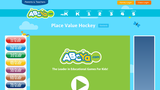Restricted Use
Rating

Place Value Hockey is a fun educational game for kids to practice ...

Place Value Hockey is a fun educational game for kids to practice place value. There are 2 modes of play and 3 levels of difficulty. Modes of play: Identify Place Value &amp; Type Numerals Difficulty: Level 1: ones to hundreds; Level 2: ones to millions; Level 3: ten thousandths to millions The game will show percentage correct and players get to take penalty shots when they get five correct in a row. You could use this resource as a formative assessment, game for kids to practice place value, or as a station in your math workshop.

Subject:
Mathematics
Material Type:
Game
Self Assessment
Provider:
ABCya.com
04/10/2017Unrestricted Use
CC BY
Rating

This lesson was used with a tier 3 fourth grade intervention math ...

This lesson was used with a tier 3 fourth grade intervention math group to help students to progress from counting by tens and ones to combining and separating by tens and ones without skip counting. I used this Illustrative Math lesson, https://www.illustrativemathematics.org/content-standards/tasks/2106, and modified it to meet the students' needs. I modified the lesson by doing only the activities in Part 3 and then extending this by modeling the equations, that matched the hundreds chart work, with base ten blocks. I also made sure students saw the pattern in adding ones and adding tens and encouraged the proper place value terms. For example, "4 + 3 = 7 so 4 groups of ten + 3 groups of ten = 7 groups of ten which is the same as 40 + 30 = 70." I then extended this by having students add numbers with tens and ones to number with tens and ones with and without regrouping and asked them to first model it on the hundreds chart and with base ten blocks and then do the addition without skip counting (first adding the tens and then the ones and then combine the results).

Subject:
Mathematics
Material Type:
Author:
MEGAN HINDE
08/02/2019Conditional Remix & Share Permitted
CC BY-NC-SA
Rating

This task serves as a bridge between understanding place-value and using strategies ...

This task serves as a bridge between understanding place-value and using strategies based on place-value structure for addition.

Subject:
Mathematics
Numbers and Operations
Material Type:
Activity/Lab
Provider:
Illustrative Mathematics
Provider Set:
Illustrative Mathematics
Author:
Illustrative Mathematics
05/01/2012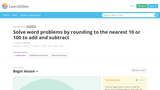Restricted Use
Rating

Big Ideas: Rounding is an appropriate strategy for solving problems and estimating. ...

Big Ideas: Rounding is an appropriate strategy for solving problems and estimating. Numbers can be decomposed and recomposed to add and subtract.
This lesson builds on student knowledge of place value of whole numbers less than or equal to 1000. This task uses a range of weights for endangered animals for students to apply rounding principles to make totals of 1000 and 2000 using addition and subtraction strategies. This builds conceptual understanding of rounding to build upon for practical use for problem solving using addition, subtraction, multiplication, and division.
Vocabulary: base-ten, expanded form, place value, accurate, complements, commutative property, partial sums

Subject:
Mathematics
Material Type:
Diagram/Illustration
Formative Assessment
Lesson Plan
Provider:
Learn Zillion
06/16/2015Conditional Remix & Share Permitted
CC BY-NC-SA
Rating

The purpose of this task is to help students understand composing and ...

The purpose of this task is to help students understand composing and decomposing ones, tens, and hundreds. This task is meant to be used in an instructional setting and would only be appropriate to use if students actually have base-ten blocks on hand.

Subject:
Mathematics
Numbers and Operations
Material Type:
Activity/Lab
Provider:
Illustrative Mathematics
Provider Set:
Illustrative Mathematics
Author:
Illustrative Mathematics